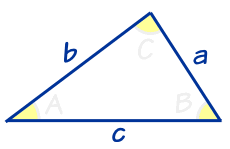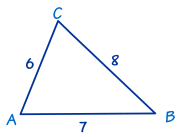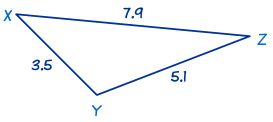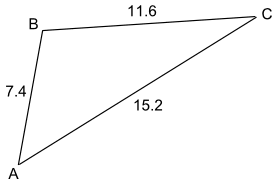# 解 SSS 三角形

"SSS" 的意思是 "(S)ide, (S)ide, (S)ide"（英语 "Angle" 的意思是 角，"Side" 的意思是 边）"SSS" 的意思是我们知道三个边长，而想求 位知的角度。

 解 SSS 三角形： 先用 余弦定理 来求其中一个角 然后再用余弦定理来求另一个角 最后用 三角形内角的和是 180° 来求最后一个角。

cos(C) = a2 + b2 − c2 2ab

cos(A) = b2 + c2 − a2 2bc

cos(B) = c2 + a2 − b2 2ca

（以上全是相同的公式，只不过标志不同）

### 例 1• a = 8，
• b = 6，
• c = 7。

cos A = (b2 + c2 − a2) / 2bc
cos A = (62 + 72 − 82) / (2×6×7)
cos A = (36 + 49 − 64) / 84
cos A = 0.25
A = cos−1(0.25)
A = 75.5224……°
A = 75.5° 准确到1个小数位。

cos B = (c2 + a2 − b2)/2ca
cos B = (72 + 82 − 62)/(2×7×8)
cos B = (49 + 64 − 36) / 112
cos B = 0.6875
B = cos−1(0.6875)
B = 46.5674……°
B = 46.6° 准确到1个小数位

C = 180° − 75.5224……° − 46.5674……°
C = 57.9° 准确到1个小数位

### 例 2cos X = (y2 + z2 − x2)/2yz
cos X = ((7.9)2 + (3.5)2 − (5.1)2)/(2×7.9×3.5)
cos X = (62.41 + 12.25 − 26.01)/55.3
cos X = 48.65/55.3 = 0.8797……
X = cos−1(0.8797……)
X = 28.3881……°
X = 28.4° 准确到1个小数位

cos Y = (z2 + x2 − y2)/2zx
cos Y = −24.15/35.7 = −0.6764……
cos Y = (12.25 + 26.01 − 62.41)/35.7
cos Y = −24.15/35.7 = −0.6764……
Y = cos−1(−0.6764……)
Y = 132.5684……°
Y = 132.6° 准确到1个小数位。

Z = 180° − 28.3881……° − 132.5684……°
Z = 19.0° 准确到1个小数位

## 另一个方法

 这是另一个（快一点的）方法来解 SSS 三角形: 先用 余弦定理 来求 最大的角 然后用 正弦定理 来求另一个角 最后用 三角形内角的和是 180° 来求最后的角。

### 例 3B 是最大的角，所以先用余弦定理来求 B：

cos B = (a2 + c2 − b2) / 2ac
cos B = (11.62 + 7.42 − 15.22) / (2×11.6×7.4)
cos B = (134.56 + 54.76 − 231.04) / 171.68
cos B = −41.72 / 171.68
cos B = −0.2430……
B = 104.1° 准确到1个小数位

sin C / 7.4 = sin 104.1° / 15.2
sin C = 7.4 × sin 104.1° / 15.2
sin C = 0.4722……
C = 28.2° 准确到1个小数位

A = 180° − (104.1° + 28.2°)
A = 180° − 132.3°
A = 47.7° 准确到1个小数位

So A = 47.7°、B = 104.1° 和 C = 28.2°